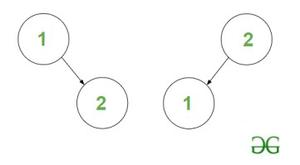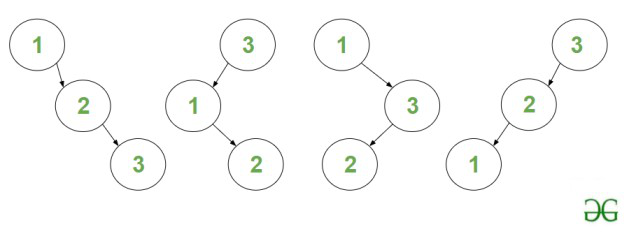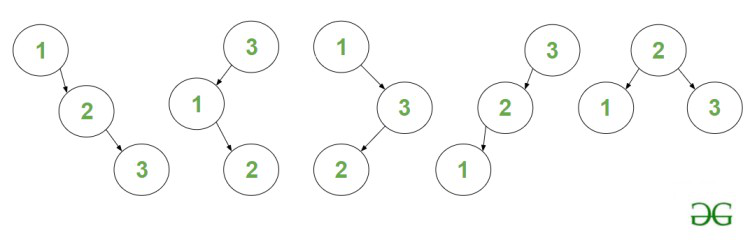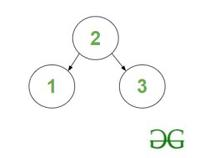Open in App
Not now

# Count of BSTs having N nodes and maximum depth equal to H

• Last Updated : 21 Mar, 2023

Given two integers N and H, the task is to find the count of distinct Binary Search Trees consisting of N nodes where the maximum depth or height of the tree is equal to H

Note: The height of BST with only the root node is 0.

Examples:

Input: N = 2, H = 1
Output: 2
Explanation: The two BST’s are :BST’s of height H = 1 and nodes N = 2

Input: N = 3, H = 2
Output: 4
Explanation: The four BST are :BST’s of height H = 2 and nodes N = 3

Naive Approach: The problem can be solved using Recursion which can be memoized to obtain a Dynamic Programming solution based on the following idea:

The problem can be efficiently solved by finding the count of BST’s having maximum depth upto H (i.e., [0 – H]) instead of exactly H.

Let f(N, H) represent the count of BST’s consisting of ‘N’ nodes and having maximum depth upto ‘H’. Then the solution for the above problem: count of BST’s having maximum depth of exactly ‘H’ is equal to f(N, H) – f(N, H – 1)

Follow the illustration below for a better understanding.

Illustration:

Consider: N = 3, H = 2

The answer for this example is : count of BST’s of maximum depth upto 2 –  count of BST’s of maximum depth upto 1.

• Count of BST’s of maximum depth upto 2 is 5, they are:5 – BST’s of maximum depth upto 2

• Count of BST’s of maximum depth upto 1 is 1, it is :1 – BST of maximum depth upto 1

• Hence the count of BST’s of maximum depth equal to ‘2’ is 4.

Follow the steps mentioned below to solve the problem.

• The count of BST with Node i as root Node is equal to product of count of BST’s of left subtree formed by nodes 1 to i-1 and right subtree formed by nodes i+1 to N.
• In order to find the count of BST of left subtree, we can recursively call the same function for depth H-1 and  N=i – 1. To find the count of BST of right subtree, recursively call the function for depth H-1 and N=N-i.
• Loop over all values of i from [1, N] as root node and add the product of count of left and right subtree to the result.

Time Complexity: O(N * 2N)
Auxiliary Space: O(1)

Efficient Approach: The above approach can be optimized by using Dynamic Programming because the above problem has Overlapping subproblems and an Optimal substructure. The subproblems can be stored in dp[][] table memoization where dp[N][H] stores the count of BST of maximum depth up to H consisting of N nodes. Follow the steps below to solve the problem:

• Initialize a global multidimensional array dp with all values as -1 that stores the result of each recursive call.
• Define a recursive function, say countOfBST(N, H) and perform the following steps.
• Case 1: If N = 0, return 1.
• Case 2: If H = 0, return true if N = 1.
• If the result of the state dp[N][H] is already computed, return this value dp[N][H].
• Iterate over the range [1, N] using the variable ‘i‘ as root and perform the following operations.
• Multiply the value of recursive functions countOfBST(i – 1, H – 1) and countOfBST(N – i, H – 1). The two functions calculate the count of BST for the left and the right subtree respectively.
• Add the term to the final answer which stores the total count of BSTs possible for all roots from [1, N].
• Print the value returned by the function countOfBST(N, H).

Below is the implementation of the above approach :

## C++

 `// C++ code to implement the approach`   `#include ` `using` `namespace` `std;`   `// Declaring a dp-array` `int` `dp;`   `const` `int` `mod = 1000000007;`   `// Function to find the count of` `// BST upto height 'H' consisting` `// of 'N' nodes.` `int` `countOfBST(``int` `N, ``int` `H)` `{`   `    ``// Base Case1 : If N == 0, return` `    ``// 1 as a valid BST has been formed` `    ``if` `(N == 0) {` `        ``return` `1;` `    ``}`   `    ``// Base Case2 : If H == 0, return true` `    ``// if N == 1` `    ``if` `(H == 0) {` `        ``return` `N == 1;` `    ``}`   `    ``// If the current state has already` `    ``// been computed, then return it.` `    ``if` `(dp[N][H] != -1) {` `        ``return` `dp[N][H];` `    ``}`   `    ``// Initialize answer to 0.` `    ``int` `ans = 0;`   `    ``// Iterate over all numbers from` `    ``// [1, N], with 'i' as root.` `    ``for` `(``int` `i = 1; i <= N; ++i) {`   `        ``// Call the recursive functions to` `        ``// find count of BST of left and right` `        ``// subtrees. Add the product of` `        ``// both terms to the answer.` `        ``ans += (countOfBST(i - 1, H - 1) * 1LL` `                ``* countOfBST(N - i, H - 1))` `               ``% mod;`   `        ``// Take modulo 1000000007` `        ``ans %= mod;` `    ``}`   `    ``// Return ans` `    ``return` `dp[N][H] = ans;` `}`   `// Utility function to find the count` `// of BST upto height 'H' consisting` `// of 'N' nodes.` `int` `UtilCountOfBST(``int` `N, ``int` `H)` `{`   `    ``// Initialize dp-array with -1.` `    ``memset``(dp, -1, ``sizeof` `dp);`   `    ``// If height is 0, return true if` `    ``// only one node is present.` `    ``if` `(H == 0) {` `        ``return` `(N == 1);` `    ``}`   `    ``// Function call.` `    ``return` `(countOfBST(N, H)` `            ``- countOfBST(N, H - 1)` `            ``+ mod)` `           ``% mod;` `}`   `// Driver code` `int` `main()` `{` `    ``// Number of nodes` `    ``int` `N = 3;`   `    ``// Height of tree` `    ``int` `H = 2;`   `    ``cout << UtilCountOfBST(N, H) << endl;` `    ``return` `0;` `}`

## Java

 `// Java implementation of above approach` `import` `java.io.*;` `import` `java.util.*;`   `class` `GFG {`   `  ``// Declaring a dp-array` `  ``static` `int``[][] dp = ``new` `int``[``105``][``105``];`   `  ``static` `int` `mod = ``1000000007``;`   `  ``// Function to find the count of` `  ``// BST upto height 'H' consisting` `  ``// of 'N' nodes.` `  ``static` `int` `countOfBST(``int` `N, ``int` `H)` `  ``{`   `    ``// Base Case1 : If N == 0, return` `    ``// 1 as a valid BST has been formed` `    ``if` `(N == ``0``) {` `      ``return` `1``;` `    ``}`   `    ``// Base Case2 : If H == 0, return true` `    ``// if N == 1` `    ``if` `(H == ``0``) {` `      ``if` `(N == ``1``)` `        ``return` `1``;` `      ``return` `0``;` `    ``}`   `    ``// If the current state has already` `    ``// been computed, then return it.` `    ``if` `(dp[N][H] != -``1``) {` `      ``return` `dp[N][H];` `    ``}`   `    ``// Initialize answer to 0.` `    ``int` `ans = ``0``;`   `    ``// Iterate over all numbers from` `    ``// [1, N], with 'i' as root.` `    ``for` `(``int` `i = ``1``; i <= N; ++i) {`   `      ``// Call the recursive functions to` `      ``// find count of BST of left and right` `      ``// subtrees. Add the product of` `      ``// both terms to the answer.` `      ``ans += (countOfBST(i - ``1``, H - ``1``)` `              ``* countOfBST(N - i, H - ``1``))` `        ``% mod;`   `      ``// Take modulo 1000000007` `      ``ans %= mod;` `    ``}`   `    ``// Return ans` `    ``dp[N][H] = ans;` `    ``return` `dp[N][H];` `  ``}`   `  ``// Utility function to find the count` `  ``// of BST upto height 'H' consisting` `  ``// of 'N' nodes.` `  ``static` `int` `UtilCountOfBST(``int` `N, ``int` `H)` `  ``{`   `    ``// Initialize dp-array with -1.` `    ``for` `(``int` `i = ``0``; i < ``105``; i++)` `      ``for` `(``int` `j = ``0``; j < ``105``; j++)` `        ``dp[i][j] = -``1``;`   `    ``// If height is 0, return true if` `    ``// only one node is present.` `    ``if` `(H == ``0``) {` `      ``if` `(N == ``1``)` `        ``return` `1``;` `      ``return` `0``;` `    ``}`   `    ``// Function call.` `    ``return` `(countOfBST(N, H) - countOfBST(N, H - ``1``)` `            ``+ mod)` `      ``% mod;` `  ``}`   `  ``// Driver Code` `  ``public` `static` `void` `main(String[] args)` `  ``{`   `    ``// Number of nodes` `    ``int` `N = ``3``;`   `    ``// Height of tree` `    ``int` `H = ``2``;`   `    ``System.out.print(UtilCountOfBST(N, H));` `  ``}` `}`   `// This code is contributed by code_hunt.`

## Python3

 `# python3 code to implement the approach`   `# Declaring a dp-array` `dp ``=` `[[``-``1` `for` `_ ``in` `range``(``105``)] ``for` `_ ``in` `range``(``105``)]`   `mod ``=` `1000000007`   `# Function to find the count of` `# BST upto height 'H' consisting` `# of 'N' nodes.` `def` `countOfBST(N, H):`   `        ``# Base Case1 : If N == 0, return` `        ``# 1 as a valid BST has been formed` `    ``if` `(N ``=``=` `0``):` `        ``return` `1`   `        ``# Base Case2 : If H == 0, return true` `        ``# if N == 1` `    ``if` `(H ``=``=` `0``):` `        ``return` `N ``=``=` `1`   `        ``# If the current state has already` `        ``# been computed, then return it.` `    ``if` `(dp[N][H] !``=` `-``1``):` `        ``return` `dp[N][H]`   `        ``# Initialize answer to 0.` `    ``ans ``=` `0`   `    ``# Iterate over all numbers from` `    ``# [1, N], with 'i' as root.` `    ``for` `i ``in` `range``(``1``, N``+``1``):`   `                ``# Call the recursive functions to` `                ``# find count of BST of left and right` `                ``# subtrees. Add the product of` `                ``# both terms to the answer.` `        ``ans ``+``=` `(countOfBST(i ``-` `1``, H ``-` `1``) ``*` `countOfBST(N ``-` `i, H ``-` `1``)) ``%` `mod`   `        ``# Take modulo 1000000007` `        ``ans ``%``=` `mod`   `        ``# Return ans` `    ``dp[N][H] ``=` `ans` `    ``return` `dp[N][H]`   `# Utility function to find the count` `# of BST upto height 'H' consisting` `# of 'N' nodes.` `def` `UtilCountOfBST(N, H):`   `        ``# Initialize dp-array with -1.`   `        ``# If height is 0, return true if` `        ``# only one node is present.` `    ``if` `(H ``=``=` `0``):` `        ``return` `(N ``=``=` `1``)`   `    ``# Function call.` `    ``return` `(countOfBST(N, H)` `            ``-` `countOfBST(N, H ``-` `1``)` `            ``+` `mod) ``%` `mod`   `# Driver code` `if` `__name__ ``=``=` `"__main__"``:`   `    ``# Number of nodes` `    ``N ``=` `3`   `    ``# Height of tree` `    ``H ``=` `2`   `    ``print``(UtilCountOfBST(N, H))`   `    ``# This code is contributed by rakeshsahni`

## C#

 `// C# code to implement the approach` `using` `System;` `class` `GFG {`   `  ``// Declaring a dp-array` `  ``static` `int``[, ] dp = ``new` `int``[105, 105];`   `  ``const` `int` `mod = 1000000007;`   `  ``// Function to find the count of` `  ``// BST upto height 'H' consisting` `  ``// of 'N' nodes.` `  ``static` `int` `countOfBST(``int` `N, ``int` `H)` `  ``{`   `    ``// Base Case1 : If N == 0, return` `    ``// 1 as a valid BST has been formed` `    ``if` `(N == 0) {` `      ``return` `1;` `    ``}`   `    ``// Base Case2 : If H == 0, return true` `    ``// if N == 1` `    ``if` `(H == 0) {` `      ``if` `(N == 1)` `        ``return` `1;` `      ``return` `0;` `    ``}`   `    ``// If the current state has already` `    ``// been computed, then return it.` `    ``if` `(dp[N, H] != -1) {` `      ``return` `dp[N, H];` `    ``}`   `    ``// Initialize answer to 0.` `    ``int` `ans = 0;`   `    ``// Iterate over all numbers from` `    ``// [1, N], with 'i' as root.` `    ``for` `(``int` `i = 1; i <= N; ++i) {`   `      ``// Call the recursive functions to` `      ``// find count of BST of left and right` `      ``// subtrees. Add the product of` `      ``// both terms to the answer.` `      ``ans += (countOfBST(i - 1, H - 1)` `              ``* countOfBST(N - i, H - 1))` `        ``% mod;`   `      ``// Take modulo 1000000007` `      ``ans %= mod;` `    ``}`   `    ``// Return ans` `    ``dp[N, H] = ans;` `    ``return` `dp[N, H];` `  ``}`   `  ``// Utility function to find the count` `  ``// of BST upto height 'H' consisting` `  ``// of 'N' nodes.` `  ``static` `int` `UtilCountOfBST(``int` `N, ``int` `H)` `  ``{`   `    ``// Initialize dp-array with -1.` `    ``for` `(``int` `i = 0; i < 105; i++)` `      ``for` `(``int` `j = 0; j < 105; j++)` `        ``dp[i, j] = -1;`   `    ``// If height is 0, return true if` `    ``// only one node is present.` `    ``if` `(H == 0) {` `      ``if` `(N == 1)` `        ``return` `1;` `      ``return` `0;` `    ``}`   `    ``// Function call.` `    ``return` `(countOfBST(N, H) - countOfBST(N, H - 1)` `            ``+ mod)` `      ``% mod;` `  ``}`   `  ``// Driver code` `  ``public` `static` `void` `Main()` `  ``{` `    ``// Number of nodes` `    ``int` `N = 3;`   `    ``// Height of tree` `    ``int` `H = 2;`   `    ``Console.Write(UtilCountOfBST(N, H));` `  ``}` `}`   `// This code is contributed by ukasp.`

## Javascript

 ``

Output

`4`

Time Complexity: O(N2 * H)
Auxiliary Space: O(N * H)

Efficient approach : Using DP Tabulation method ( Iterative approach )

The approach to solve this problem is same but DP tabulation(bottom-up) method is better then Dp + memorization(top-down) because memorization method needs extra stack space of recursion calls.

Steps to solve this problem :

• Create a table to store the solution of the subproblems.
• Initialize the table with base cases
• Fill up the table iteratively
• Return the final solution

Implementation :

## C++

 `// C++ program for the above approach` `#include ` `using` `namespace` `std;`   `const` `int` `mod = 1000000007;`   `// Function to find the count of` `// BST upto height 'H' consisting` `// of 'N' nodes.` `int` `countOfBST(``int` `N, ``int` `H)` `{` `    ``// Initialize dp-array` `    ``int` `dp[N + 1][H + 1];` `    ``memset``(dp, 0, ``sizeof``(dp));`   `    ``// Base Case1 : If N == 0, return` `    ``// 1 as a valid BST has been formed` `    ``for` `(``int` `i = 0; i <= H; ++i) {` `        ``dp[i] = 1;` `    ``}`   `    ``// Base Case2 : If H == 0, return true` `    ``// if N == 1` `    ``for` `(``int` `i = 1; i <= N; ++i) {` `        ``dp[i] = (i == 1);` `    ``}`   `    ``// Iterate over all nodes and height` `    ``for` `(``int` `i = 1; i <= N; ++i) {` `        ``for` `(``int` `j = 1; j <= H; ++j) {` `            ``for` `(``int` `k = 1; k <= i; ++k) {` `                ``dp[i][j] = (dp[i][j] +` `                            ``(dp[k - 1][j - 1] * 1LL * dp[i - k][j - 1]) % mod) %` `                           ``mod;` `            ``}` `        ``}` `    ``}`   `    ``// Return ans` `    ``return` `dp[N][H];` `}`   `// Utility function to find the count` `// of BST upto height 'H' consisting` `// of 'N' nodes.` `int` `UtilCountOfBST(``int` `N, ``int` `H)` `{` `    ``if` `(H == 0) {` `        ``return` `(N == 1);` `    ``}`   `    ``// Function call.` `    ``return` `((countOfBST(N, H) - countOfBST(N, H - 1) + mod) % mod);` `}`   `// Driver code` `int` `main()` `{` `    ``// Number of nodes` `    ``int` `N = 3;`   `    ``// Height of tree` `    ``int` `H = 2;`   `    ``cout << UtilCountOfBST(N, H) << endl;` `    ``return` `0;` `}`   `// this code is contributed by bhardwajji`

## Python3

 `# Python program for the above approach` `MOD ``=` `1000000007`   `# Function to find the count of` `# BST upto height 'H' consisting` `# of 'N' nodes.` `def` `countOfBST(N: ``int``, H: ``int``) ``-``> ``int``:` `    ``# Initialize dp-array` `    ``dp ``=` `[[``0` `for` `j ``in` `range``(H``+``1``)] ``for` `i ``in` `range``(N``+``1``)]`   `    ``# Base Case1 : If N == 0, return` `    ``# 1 as a valid BST has been formed` `    ``for` `i ``in` `range``(H``+``1``):` `        ``dp[``0``][i] ``=` `1`   `    ``# Base Case2 : If H == 0, return true` `    ``# if N == 1` `    ``for` `i ``in` `range``(``1``, N``+``1``):` `        ``dp[i][``0``] ``=` `1` `if` `i ``=``=` `1` `else` `0`   `    ``# Iterate over all nodes and height` `    ``for` `i ``in` `range``(``1``, N``+``1``):` `        ``for` `j ``in` `range``(``1``, H``+``1``):` `            ``for` `k ``in` `range``(``1``, i``+``1``):` `                ``dp[i][j] ``=` `(dp[i][j] ``+` `                            ``(dp[k ``-` `1``][j ``-` `1``] ``*` `dp[i ``-` `k][j ``-` `1``]) ``%` `MOD) ``%` `MOD`   `    ``# Return ans` `    ``return` `dp[N][H]`   `# Utility function to find the count` `# of BST upto height 'H' consisting` `# of 'N' nodes.` `def` `UtilCountOfBST(N: ``int``, H: ``int``) ``-``> ``int``:` `    ``if` `H ``=``=` `0``:` `        ``return` `1` `if` `N ``=``=` `1` `else` `0`   `    ``# Function call.` `    ``return` `((countOfBST(N, H) ``-` `countOfBST(N, H ``-` `1``) ``+` `MOD) ``%` `MOD)`   `# Driver code` `if` `__name__ ``=``=` `"__main__"``:` `    ``# Number of nodes` `    ``N ``=` `3`   `    ``# Height of tree` `    ``H ``=` `2`   `    ``print``(UtilCountOfBST(N, H))`

Output :

`4`

Time Complexity: O(N2 * H)
Auxiliary Space: O(N * H)

My Personal Notes arrow_drop_up
Related Articles